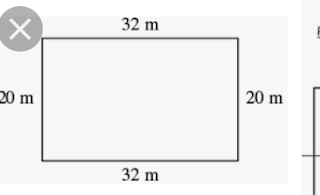## Sunday, January 27, 2019

MATHEMATICS

SECOND TERM

WEEK 4

PRIMARY 6

TOPIC: Perimeter

LEARNING AREA

Lesson One - Introduction to perimeter
Lesson Two - Perimeter of rectangle
Lesson Three - Perimeter of compound shapes
Lesson Four - Quantitative Reasoning
TIME - 40 Minutes each.

LEARNING OUTCOME

By the end of the lesson, the pupils should be able to:

Reviewing work done on perimeters of plane shapes.

Identifying rectangles that have same area but different perimeters.

Finding the perimeters of compound shapes.
RATIONALE

Perimeter is a distance round a shape.

Perimeter means the sum of lengths of all the sides of a plane shape - triangles, other quadrilaterals and higher polygons = sum of lengths of all sides. It can be used to calculate the length of fence surrounding a building or garden. Two shapes may have the same perimeter, but different areas or may have the same area, but different perimeters.The concept of perimeter will enable pupils to understand the different between areas and perimeter and appreciate the use of perimeter within and around us.

LEARNING MATERIALS

Ruler and measuring tape
Box - square and rectangular faces
Chart of triangle, square and rectangle
Improvises measuring materials like hands, legs, or any length wood or rod.
PREVIOUS KNOWLEDGE

Measuring length
Triangle
Square
Rectangle
Area of triangle, square and rectangle.
REFERENCE MATERIALS

9 - Years Basic Education Curriculum
Lagos State Scheme of work
New Method Mathematics Book 6
All Relevant Materials
Online Materials
LESSON PLAN/DEVELOPMENT

LESSON DEVELOPMENT 1 - INTRODUCTION OF PERIMETER document

Step 1 - Introduction (5 minutes)

TEACHER'S ACTIVITIES

Guides pupils to identify different types of plane shapes on chart.
PUPIL'S ACTIVITIES

Pupils identify triangle (types), square, rectangle, etc..
LEARNING POINT - Previous knowledge and lesson

Step 2 - Development (5 minutes)

Groups the pupils into four groups – A, B, C, and D.
Guide the pupils to choose a leader and secretary for your group.
Gives each group learning materials – measuring tape.
PUPIL'S ACTIVITIES

Belong to a group.
Choose their leader and secretary.
Received learning materials for their group.
LEARNING POINT - Pupil's group, leader and secretary confirmed.

Step 3 -Development - (10 minutes)

TEACHER'S ACTIVITIES

Takes pupils out to the field and guides them to measure the lengths of the field.
Names each length according to their group – length A, B C and D.
PUPIL'S ACTIVITIES

Measure the lengths of the School's field.
Length A -
Length B -
Length C -
Length D -
LEARNING POINT - Length

Step 4 -Development - (10 minutes)

Guides the pupils to add –

Length (A + B), named it AB
Length (C + D), named it CD
Length (AB + CD), named it perimeter.
Pupil's Activities

Working

Length AB = ____ cm
Length CD = ____ cm
Perimeter = ____ cm
LEARNING POINT - Introduction to perimeter

Step 5 - CONCLUSION (10 minutes)

CONCLUSION

To conclude the lesson for the week, the teacher revises (through questions and answers) the entire lesson and links it to the next lesson.

PUPIL'S ACTIVITIESListen, ask and answer questions.
Perimeter means the sum of lengths all the sides of a plane shape.
LEARNING POINT - Conclusion

LESSON DEVELOPMENT 2 - PERIMETER OF RECTANGLE

LESSON DEVELOPMENT 3 - PERIMETER OF COMPOUND SHAPES